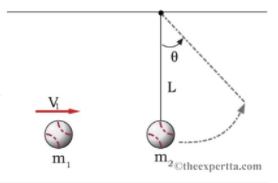# Problem: A baseball of mass m1 = 0.37 kg is thrown at another ball hanging from the ceiling by a length of string L = 1.45 m. The second ball m2 = 0.72 kg is initially at rest while the baseball has an initial horizontal velocity of V1 = 4.5 m/s. After the collision the first baseball falls straight down (no horizontal velocity.) m1 = 0.37 kgm2 = 0.72 kgL = 1.45 mV1 = 4.5 m/sPart (a) Select an expression for the magnitude of the closest distance from the ceiling the second ball will reach d. Schematic Choice:a) d=(m1V1)22m22gb) d=L-V122gc) d=L-m1V12m2gd) d=L-(m1V1)22m22gPart (b) What is the angle that the string makes with the vertical at the highest point of travel in degrees? Numeric: A numeric value is expected and not an expression.θ = _____________

###### FREE Expert Solution

In this problem, we have collisions, kinetic, and gravitational potential energy.

Conservation of momentum:

$\overline{){{\mathbf{m}}}_{{\mathbf{1}}}{{\mathbf{v}}}_{{\mathbf{01}}}{\mathbf{+}}{{\mathbf{m}}}_{{\mathbf{2}}}{{\mathbf{v}}}_{{\mathbf{02}}}{\mathbf{=}}{{\mathbf{m}}}_{{\mathbf{1}}}{{\mathbf{v}}}_{\mathbf{f}\mathbf{1}}{\mathbf{+}}{{\mathbf{m}}}_{{\mathbf{2}}}{{\mathbf{v}}}_{\mathbf{f}\mathbf{2}}}$

Conservation of energy:

$\overline{){{\mathbf{K}}}_{{\mathbf{0}}}{\mathbf{+}}{{\mathbf{U}}}_{{\mathbf{0}}}{\mathbf{+}}{{\mathbf{W}}}_{{\mathbf{nc}}}{\mathbf{=}}{{\mathbf{K}}}_{{\mathbf{f}}}{\mathbf{+}}{{\mathbf{U}}}_{{\mathbf{f}}}}$ where Wnc is the work done by non-conservative forces such as friction.

Height of pendulum:

$\overline{){\mathbf{h}}{\mathbf{=}}{\mathbf{L}}{\mathbf{-}}{\mathbf{L}}{\mathbf{c}}{\mathbf{o}}{\mathbf{s}}{\mathbf{\theta }}}$

92% (405 ratings)###### Problem DetailsA baseball of mass m1 = 0.37 kg is thrown at another ball hanging from the ceiling by a length of string L = 1.45 m. The second ball m2 = 0.72 kg is initially at rest while the baseball has an initial horizontal velocity of V1 = 4.5 m/s. After the collision the first baseball falls straight down (no horizontal velocity.)

m1 = 0.37 kg
m2 = 0.72 kg
L = 1.45 m
V1 = 4.5 m/s

Part (a) Select an expression for the magnitude of the closest distance from the ceiling the second ball will reach d

Schematic Choice:

Part (b) What is the angle that the string makes with the vertical at the highest point of travel in degrees? Numeric: A numeric value is expected and not an expression.

θ = _____________

Frequently Asked Questions

What scientific concept do you need to know in order to solve this problem?

Our tutors have indicated that to solve this problem you will need to apply the Collisions & Motion (Momentum & Energy) concept. You can view video lessons to learn Collisions & Motion (Momentum & Energy). Or if you need more Collisions & Motion (Momentum & Energy) practice, you can also practice Collisions & Motion (Momentum & Energy) practice problems.